Question

Let the Sample Space S be defined as equally likely integer values from 2 to 18 (2, 3, 4, 5, 6, 7, 8, 9, 10, 11, 12, 13, 14, 15, 16, 17, 18). Also, let event A be defined as (2, 3, 4, 5, 6, 7) and event B as (6, 7, 9, 10).

a) What is the conditional probability P(B|A)?

b) What is the probability P(A ∪ B)?

a) n(A) = 6

n(B|A) = n( {6,7} ) = 2

The conditional probability P(B|A)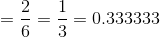b) A U B = { 2,3,4,5,6,7,9,10}

n(A U B) = 8

and n(S) = 17

The  probability P(A ∪ B) is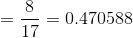#### Earn Coins

Coins can be redeemed for fabulous gifts.

Similar Homework Help Questions
• ### A probability experiment is conducted in which the sample space of the experiment is S={7, 8,...

A probability experiment is conducted in which the sample space of the experiment is S={7, 8, 9, 10, 11, 12, 13, 14, 15, 16, 17, 18}, event F= {10,11,12,13,14}, and event G={14, 15, 16, 17}. Assume that each outcome is equally likely. List the outcomes in F or G. Find P(F or G) by counting the number of outcomes in F or G. Determine P(F or G) using the general addition rule.

• ### Let the sample space be S = {1,2,3,4,5,6,7,8,9,10). Suppose the outcomes are equally likely. Compute the...Let the sample space be S = {1,2,3,4,5,6,7,8,9,10). Suppose the outcomes are equally likely. Compute the probability of the event E="an odd number less than 7." PE=(Type an integer or a decimal. Do not round.) ook arce otes vity Enter your answer in the answer box course summer based on a normed versions using Data,

• ### A probability experiment is conducted in which the sample space of the experiment is S 1,2,...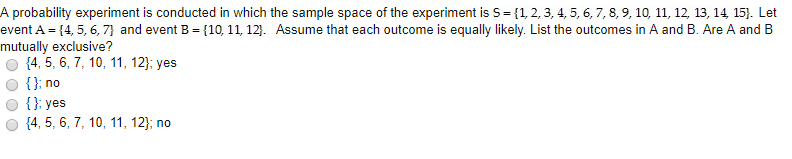A probability experiment is conducted in which the sample space of the experiment is S 1,2, 3, 4,5, 6,7, 8, 9, 10, 11, 12, 13, 14, 15]. Let event A = {4, 5, 6.7 and event B = {10, 11, 12]. Assume that each outcome is equally likely. List the outcomes in A and B. Are A and B mutually exclusive? 14,5, 6,7, 10, 11, 12): yes Ono O () yes 14, 5,6, 7, 10, 11, 12,no

• ### Let the sample space be Upper S equals StartSet 1 comma 2 comma 3 comma 4 comma 5 comma 6 comma 7 comma 8 comma 9 comm...

Let the sample space be Upper S equals StartSet 1 comma 2 comma 3 comma 4 comma 5 comma 6 comma 7 comma 8 comma 9 comma 10 EndSetS={1, 2, 3, 4, 5, 6, 7, 8, 9, 10}. Suppose the outcomes are equally likely. Compute the probability of the event Upper E equals StartSet 1 comma 2 comma 4 comma 6 EndSetE={1, 2, 4, 6}.

• ### A probability experiment is conducted in which the sample space of the experiment is S {8,...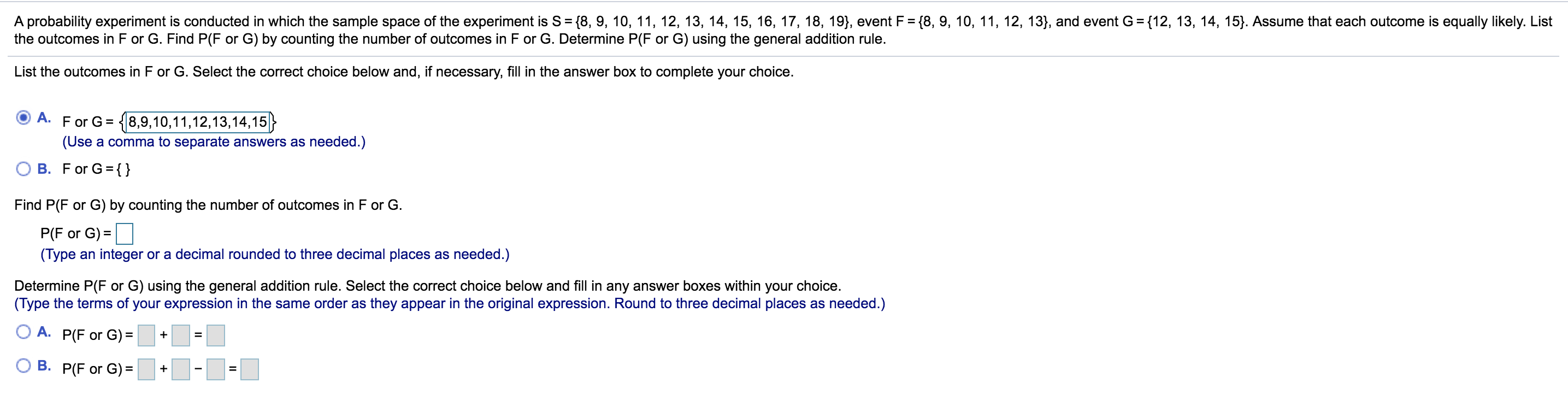A probability experiment is conducted in which the sample space of the experiment is S {8, 9, 10, 11, 12, 13, 14, 15, 16, 17, 18, 19}, event F {8, 9, 10, 11, 12, 13}, and event G {12, 13, 14, 15}. Assume that each outcome is equally likely. List the outcomes in F or G. Find P(F or G) by counting the number of outcomes in F or G. Determine P(F or G) using the general addition rule. List...

• ### A probability experimentis conducted in which the sample space of the experimentis S =(2, 3, 4,...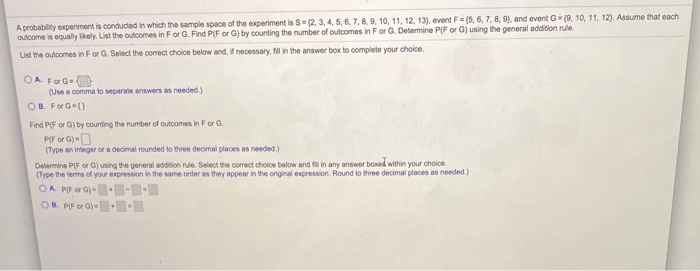A probability experimentis conducted in which the sample space of the experimentis S =(2, 3, 4, 5, 6, 7, 8, 9, 10, 11, 12, 13), event F = 15, 6, 7, 8, 9), and event G-19, 10, 11, 12). Assume that each outcome is equally likely List the outcomes in For G. Find PF or G) by counting the number of outcomes in For G. Determine P/F or G) using the general addition rule List the outcomes in For G....

• ### A probability experiment is conducted in which the sample space of the outcome is equally likely....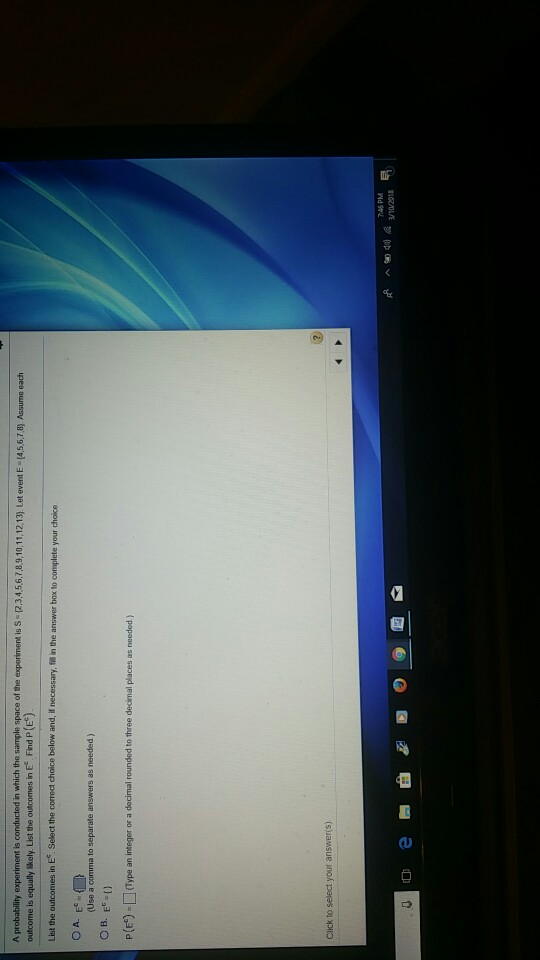A probability experiment is conducted in which the sample space of the outcome is equally likely. List the outcomes in Ec Find P(EC) List the outcomes in E. Select the correct choice below and, if necessary, fill in the answer box to complete your choice as needed) P(E) Type an integer or a decimal rounded to three decimal places as needed) Click to select your answeris) 745 PM /10/2018 ^ 44

• ### Are all disciplines in the University equally boring or there are some more boring than others?  To...

Are all disciplines in the University equally boring or there are some more boring than others?  To answer that question, a study performed at Columbia University counted the number of times per 5-minute interval when professors from three different departments said “uh” or “ah” during lectures to fill gaps between words.  These counts were used as a proxy (approximation) for the measure of class boredom.  The data from observing one hundred of 5-minute intervals from each of three departments’ professors were recorded in...

• ### 7). a.b. Let the sample space be S = {1, 2, 3, 4, 5, 6, 7,...7). a.b. Let the sample space be S = {1, 2, 3, 4, 5, 6, 7, 8, 9, 10}. Suppose the outcomes are equally likely. Compute the probability of the event E="an odd number less than 7." P(E)= (Type an integer or a decimal. Do not round.) The data in the following table show the association between cigar smoking and death from cancer for 139,625 men. Note: current cigar smoker means cigar smoker at time of death. Click the icon...

• ### 2). a.b. A probability experiment is conducted in which the sample space of the experiment is...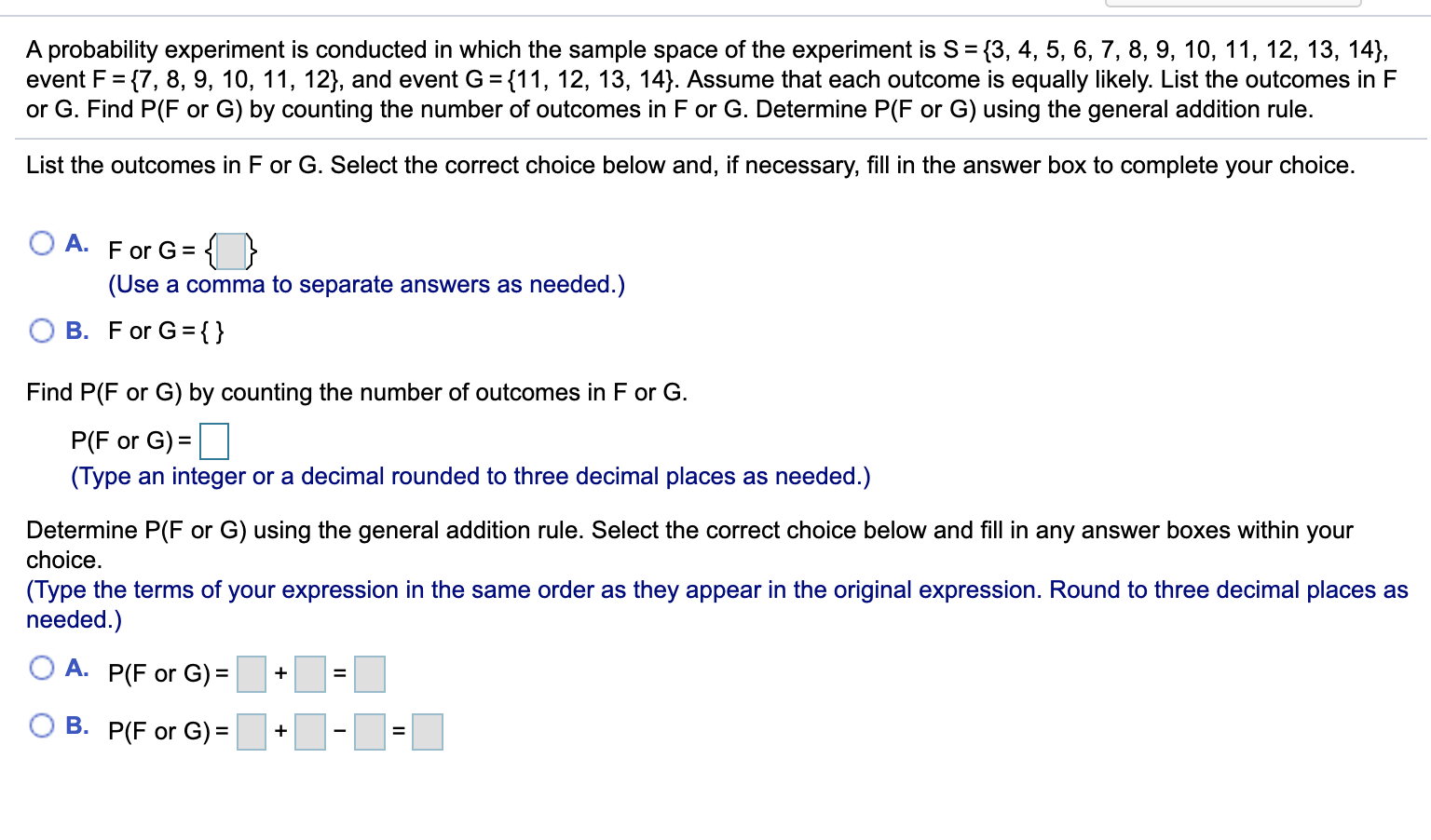2). a.b. A probability experiment is conducted in which the sample space of the experiment is S = {3, 4, 5, 6, 7, 8, 9, 10, 11, 12, 13, 14}, event F= {7, 8, 9, 10, 11, 12}, and event G = {11, 12, 13, 14). Assume that each outcome is equally likely. List the outcomes in F or G. Find P(F or G) by counting the number of outcomes in F or G. Determine P(F or G) using the...